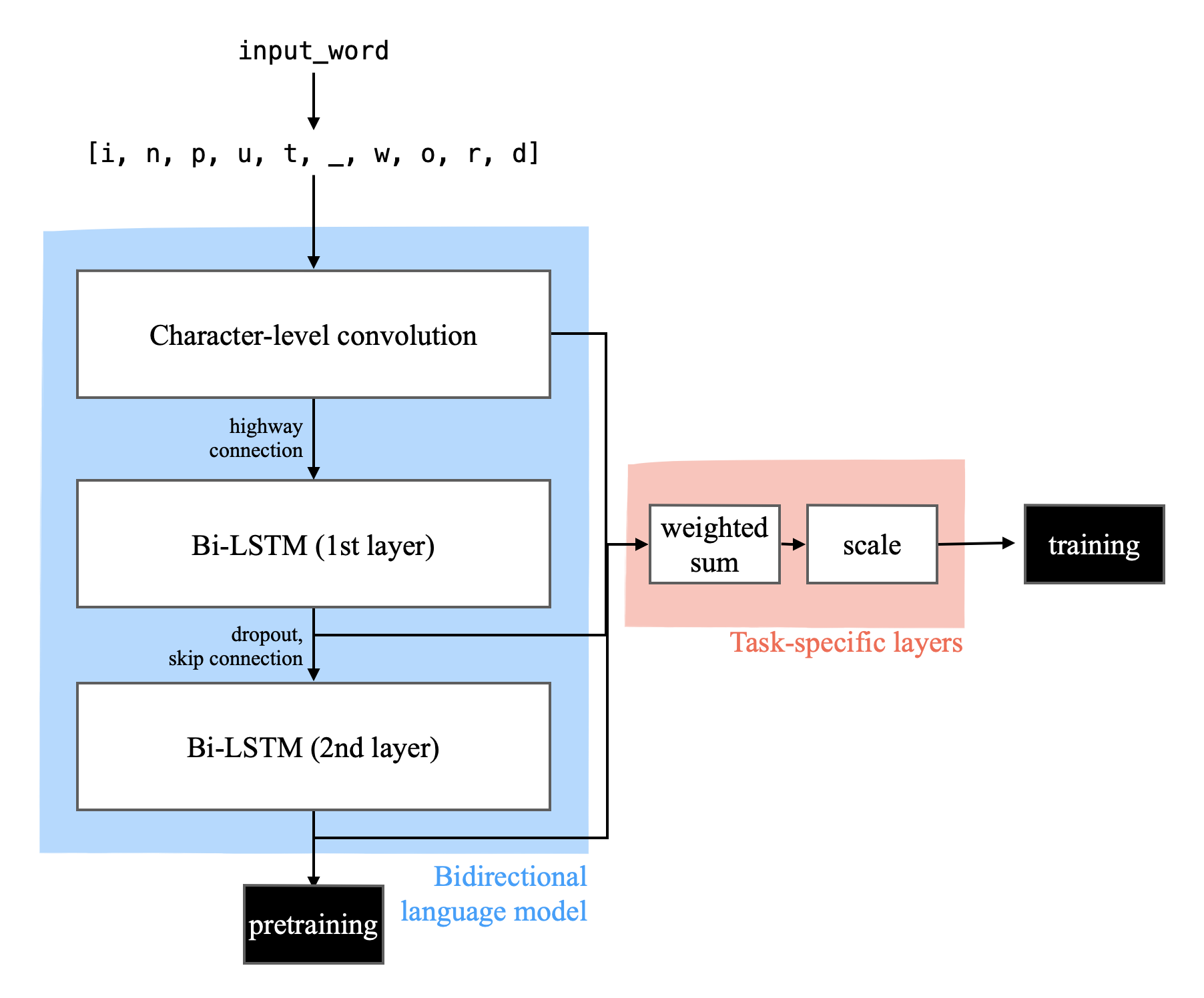# Understanding ELMo

$\newcommand{\argmin}{\mathop{\mathrm{argmin}}\limits}$ $\newcommand{\argmax}{\mathop{\mathrm{argmax}}\limits}$

Word2Vec and FastText paved the way to quality word embedding by utilizing context information, either word-level or character-level. ELMo (embeddings from language model) improved upon those with not only single context, but with both character and word-level contexts by dedicated architecture for the tasks.

ELMo is composed of two structures: bidirectional language model (biLM) and the task-specific layer. Pretrained on large data, BiLM provides enough context to task-specific layer that facilitates hi-quality embedding.

## The architecture

### Bidirectional language model

#### Character-level CNN

character-level tokens goes through convolutional layers with different kernel sizes. The original “small” ELMo model uses kernels of size 1, 2, 3, 4, 5, 6, 7 with 32, 32, 64, 128, 256, 512, 1024 channels, respectively. Outputs from each convolutional layers are then max-pooled and concatenated to yield $32+32+64+128+256+512+1024=2048$-length vector. This concatenated vector can be used as a word embedding. Since convolutional layer is well known for its feature-extracting property, this can be regarded as a character-level context extraction process.

#### Bidirectional LSTM

$L$-layer bi-LSTM is used to account for word/sentence-level information. Originally $L=2$ is used for ELMo. By using bidirectional LSTM, we can efficiently train the language model to encode contexts from the full sentence to embeddings.

One thing to note is that unlike common bi-LSTM, the one that used in ELMo separates connections between forward and backward LSTM. After the input is processed separately in each LSTM layer in each direction, only then the resulting vectors are concatenated.

According to the citation of the authors of ELMo, output from the first layer is reported to produce better result when used for POS tagging (Belinkov et al., 2017), while output from the top most layer (here, the second layer) was known for learning word-sense representations (Melamud et al., 2016).### Task specific layer

Task specific layer is a mere weighted sum and scaling of biLM outputs. All intermediate outputs from pretrained biLM, from character-level CNN and each layer of bi-LSTM, is used to train task specific layer.

Output from this layer can be further passed to other layers for downstream task such as classification. We train the whole model after freezing the pretrained biLM weights.

## Pytorch implementation

Here I pretrained the biLM using IMDB data in order to further use the pretrained model to sentiment analysis, which is positive/negative binary classification in this case.

### Character-level CNN

class CharConv(nn.Module):

def __init__(self):
super(CharConv, self).__init__()

# Embedding layer
self.char_embedding = nn.Embedding(CHAR_VOCAB_SIZE, CHAR_EMBED_DIM)

# Conv layers
self.conv1 = nn.Conv2d(CHAR_EMBED_DIM, 2, 1)
self.conv2 = nn.Conv2d(CHAR_EMBED_DIM, 2, (1, 2))
self.conv3 = nn.Conv2d(CHAR_EMBED_DIM, 4, (1, 3))
self.conv4 = nn.Conv2d(CHAR_EMBED_DIM, 8, (1, 4))
self.conv5 = nn.Conv2d(CHAR_EMBED_DIM, 16, (1, 5))
self.conv6 = nn.Conv2d(CHAR_EMBED_DIM, 32, (1, 6))
self.conv7 = nn.Conv2d(CHAR_EMBED_DIM, 64, (1, 7))
self.convs = [
self.conv1, self.conv2,
self.conv3, self.conv4,
self.conv5, self.conv6,
self.conv7,
]

def forward(self, x):
# character-level convolution
x = self.char_embedding(x).permute(0,3,1,2)
x = [conv(x) for conv in self.convs]
x = [F.max_pool2d(x_c, kernel_size=(1, x_c.shape)) for x_c in x]
x = [torch.squeeze(x_p, dim=3) for x_p in x]
x = torch.hstack(x)  # 1, n_batch, concat_length

return x


I used smaller numbers of channels, even compared to the “small” model. So final output from CharConv will be only 128-length vector per sample.

### Bidirectional LSTM

class BiLSTM(nn.Module):
def __init__(self):
super(BiLSTM, self).__init__()
# Bi-LSTM
self.lstm_f1 = nn.LSTM(128, 128)
self.lstm_r1 = nn.LSTM(128, 128)
self.dropout = nn.Dropout(0.1)
self.proj = nn.Linear(128, 64, bias=False)
self.lstm_f2 = nn.LSTM(64, 128)
self.lstm_r2 = nn.LSTM(64, 128)

def forward(self, x):
## input shape:
# seq_len, batch_size, 128

# 1st LSTM layer
x_f = x
x_r = x.flip(dims=)
## forward feed
o_f1, (h_f1, __) = self.lstm_f1(x_f)
o_f1 = self.dropout(o_f1)
## backward feed
o_r1, (h_r1, __) = self.lstm_r1(x_r)
o_r1 = self.dropout(o_r1)
h1 = torch.stack((h_f1, h_r1)).squeeze(dim=1)

# main + skip connection
x2_f = self.proj(o_f1 + x_f)
x2_r = self.proj(o_r1 + x_r)

# 2nd LSTM layer
_, (h_f2, __) = self.lstm_f2(x2_f)
_, (h_r2, __) = self.lstm_r2(x2_r)
h2 = torch.stack((h_f2, h_r2)).squeeze(dim=1)

return h1, h2


Note that feeding and forwarding into each direction is processed separately. Return from both LSTM layers were preserved for later use.

### Bidirectional language model

Stack CharConv on top of BiLM to build a biLM module.

class BiLangModel(nn.Module):
"""
Bidirectional language model (will be pretrained)
"""
def __init__(self, char_cnn, bi_lstm):
super(BiLangModel, self).__init__()

# Highway connection
self.highway = nn.Linear(128, 128)
self.transform = nn.Linear(128, 128)
self.char_cnn = char_cnn
self.bi_lstm = bi_lstm

def forward(self, x):
# Character-level convolution
x = self.char_cnn(x)
x = x.permute(2, 0, 1)

# highway
h = self.highway(x)
t_gate = torch.sigmoid(self.transform(x))
c_gate = 1 - t_gate
x_ = h * t_gate + x * c_gate

# Bi-LSTM
x1, x2 = self.bi_lstm(x_)

return x, x1, x2


Although I did not mention it before, there is in fact a highway connection between character-level CNN and bi-LSTM. BiLangModel returns all three outputs from intermediate layers (character-level CNN and two bi-LSTM layers).

[TBD: task specific layer and results]

Python code for the algorithm is in the last part of this notebook (GitHub).

References

• Peters et al. 2018. Deep contextualized word representations. https://arxiv.org/abs/1802.05365
• Network paramters were tweaked from “small” ELMo model.